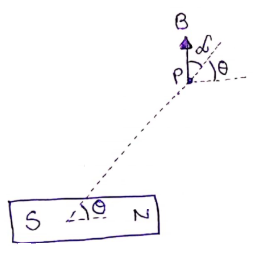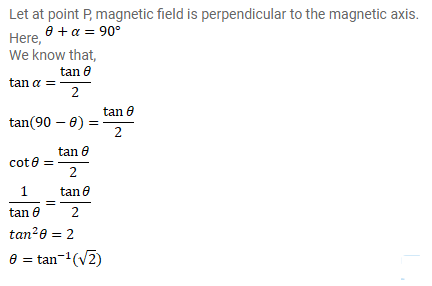# Show that the magnetic field at a point due to a magnetic dipole isQuestion:

Show that the magnetic field at a point due to a magnetic dipole is perpendicular to the magnetic axis if the line joining the point with the center of the dipole makes an angle of $\tan ^{-1}(\sqrt{2})$ with the magnetic axis.

Solution: